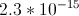# MCAT Physical : Thermodynamics of Phase Changes

## Example Questions

2 Next →

### Example Question #13 : Phase Changes

The molar heats of fusion and vaporization for water are given below: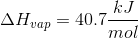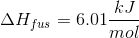Which of the following explains why the heat of vaporization is much greater than the heat of fusion?

Vaporization is an endothermic process while fusion is an exothermic process

Liquids are in constant motion and hold more energy than solids

More heat is required to vaporize a given quantity of water

The difference in kinetic energy of gas and liquid molecules is higher than the difference between solid and liquid molecules

The difference in kinetic energy of gas and liquid molecules is higher than the difference between solid and liquid molecules

Explanation:

Notice that most of these are true statements (the only incorrect statement is that fusion is an exothermic process). The key is finding the statement that best explains why the heat of vaporization is greater than the heat of fusion. Essentially, we are looking for the reason why a transition from liquid to gas requires more energy than a transition from solid to liquid.

It is true that more heat is required to vaporize a given quantity of water, but the reason for this can be found on a molecular level. Gas molecules have a relatively high kinetic energy. The large difference in kinetic energies between the states accounts for the difference in heats of fusion and vaporization. Solids are the lowest energy state, followed by liquids, and then gases. The difference in energy between equal amounts of solid and liquid is given by the heat of fusion, while the difference in energy between equal amounts of liquid and gas is given by the heat of vaporization. The discrepancy in energy is reflected in the difference between these two heat quantities.

### Example Question #11 : Phase Changes

A scientist prepares an experiment to demonstrate the second law of thermodynamics for a chemistry class. In order to conduct the experiment, the scientist brings the class outside in January and gathers a cup of water and a portable stove.

The temperature outside is –10 degrees Celsius. The scientist asks the students to consider the following when answering his questions:

Gibbs Free Energy Formula:

ΔG = ΔH – TΔS

Liquid-Solid Water Phase Change Reaction:

H2O(l) ⇌ H2O(s) + X

The scientist prepares two scenarios.

Scenario 1:

The scientist buries the cup of water outside in the snow, returns to the classroom with his class for one hour, and the class then checks on the cup. They find that the water has frozen in the cup.

Scenario 2:

The scientist then places the frozen cup of water on the stove and starts the gas. The class finds that the water melts quickly.

After the water melts, the scientist asks the students to consider two hypothetical scenarios as a thought experiment.

Scenario 3:

Once the liquid water at the end of scenario 2 melts completely, the scientist turns off the gas and monitors what happens to the water. Despite being in the cold air, the water never freezes.

Scenario 4:

The scientist takes the frozen water from the end of scenario 1, puts it on the active stove, and the water remains frozen.

In scenario 1, the Gibbs Free Energy and Keq of the Liquid-Solid Water Phase Change Reaction, as the reaction begins, is best characterized as __________.

undefined and decreasing, respectively

negative and decreasing, respectively

zero and decreasing, respectively

negative and increasing, respectively

positive and increasing, respectively

negative and increasing, respectively

Explanation:

As scenario 1 begins, the reaction is spontaneous as written, and so the Gibbs Free Energy is negative. Additionally, the Van't Hoff equation proves that Keq increases with decreasing temperature in exothermic reactions. As this reaction is exothermic and placed in low-temperature conditions, the relative abundance of the products will become the prevailing state.  Keq, therefore, increases.

### Example Question #21 : Phase Changes

Imagine a graph that shows the amount of heat being added to water. Heat (q) is on the x-axis, and the temperature of the water is on the y-axis. As more heat is added to the water, the temperature will increase, however, there are two parts of the graph that appear to plateau, and it takes a set amount of heat in order to continue raising the water's temperature.

What is the explanation for these plateaus on the graph?

It eventually requires a greater amount of heat to raise the temperature of water every Kelvin unit.

Water gives off heat very quickly, and a great amount of heat must be accumulated before the water temperature will continue to rise.

Heat is dedicated to breaking the hydrogen bonds between water molecules at these plateaus.

Water expands when heated, and the heat must warm a larger area. This results in a temporary plateau in temperature increase.

Heat is dedicated to breaking the hydrogen bonds between water molecules at these plateaus.

Explanation:

There are two plateaus on the graph, at 273K and 373K. These are the melting point and boiling points of water, respectively. At these temperatures, heat is responsible for breaking the hydrogen bonds between molecules in order to cause the phase change. The amount of heat required to melt the ice is called the enthalpy of fusion, and the amount of heat necessary to vaporize water is called the enthalpy of vaporization.

### Example Question #11 : Thermodynamics Of Phase Changes

A scientist prepares an experiment to demonstrate the second law of thermodynamics for a chemistry class. In order to conduct the experiment, the scientist brings the class outside in January and gathers a cup of water and a portable stove.

The temperature outside is –10 degrees Celsius. The scientist asks the students to consider the following when answering his questions:

Gibbs Free Energy Formula:

ΔG = ΔH – TΔS

Liquid-Solid Water Phase Change Reaction:

H2O(l) ⇌ H2O(s) + X

The scientist prepares two scenarios.

Scenario 1:

The scientist buries the cup of water outside in the snow, returns to the classroom with his class for one hour, and the class then checks on the cup. They find that the water has frozen in the cup.

Scenario 2:

The scientist then places the frozen cup of water on the stove and starts the gas. The class finds that the water melts quickly.

After the water melts, the scientist asks the students to consider two hypothetical scenarios as a thought experiment.

Scenario 3:

Once the liquid water at the end of scenario 2 melts completely, the scientist turns off the gas and monitors what happens to the water. Despite being in the cold air, the water never freezes.

Scenario 4:

The scientist takes the frozen water from the end of scenario 1, puts it on the active stove, and the water remains frozen.

When the scientist moves the frozen water to the stove, the water melts. If the temperature of the stove is kept such that the reaction is at equilibrium, and the water is almost entirely melted, which of the following is a possible Keq value for the Liquid-Solid Water Phase Change Reaction as it is written?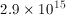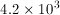0

1